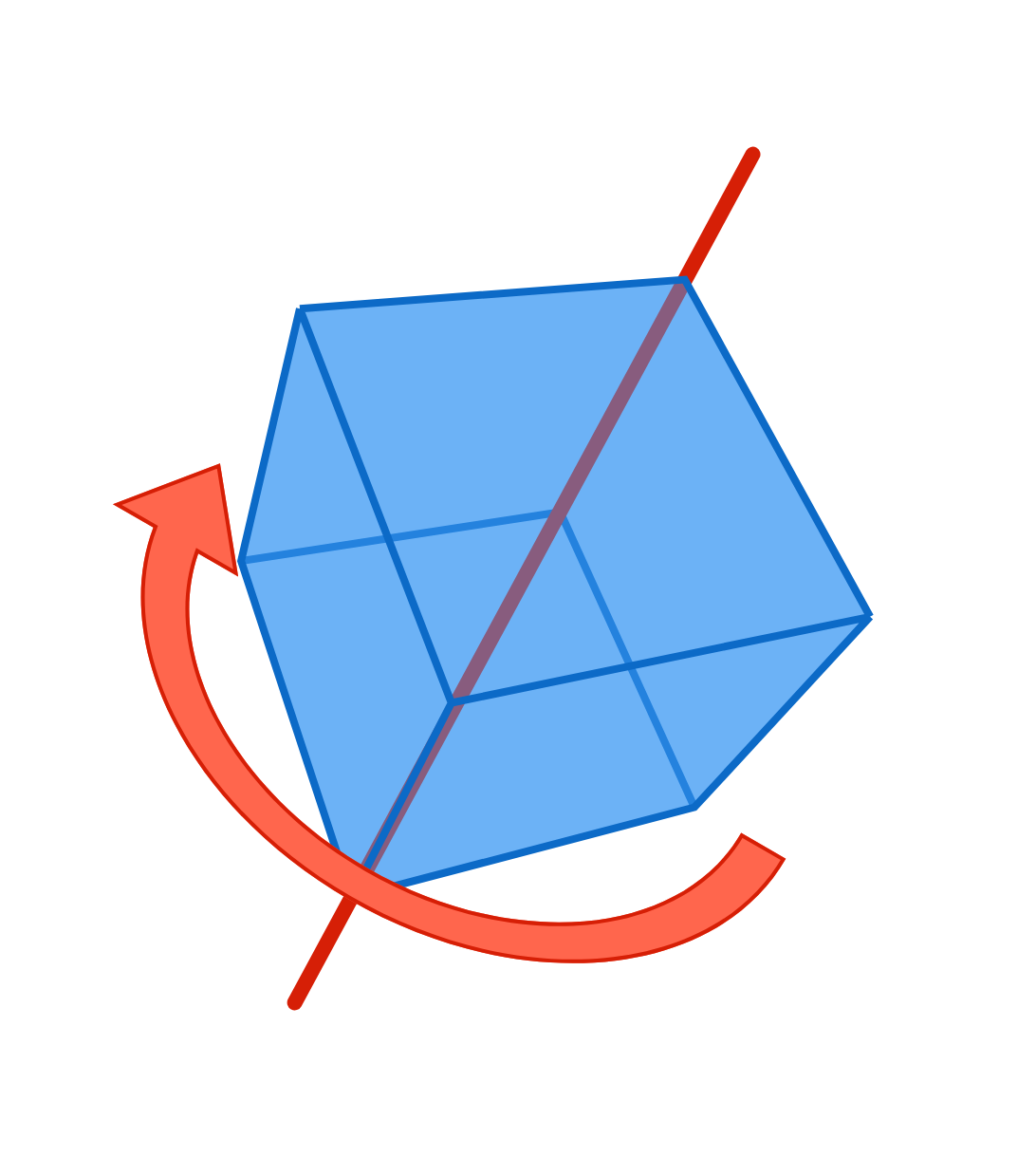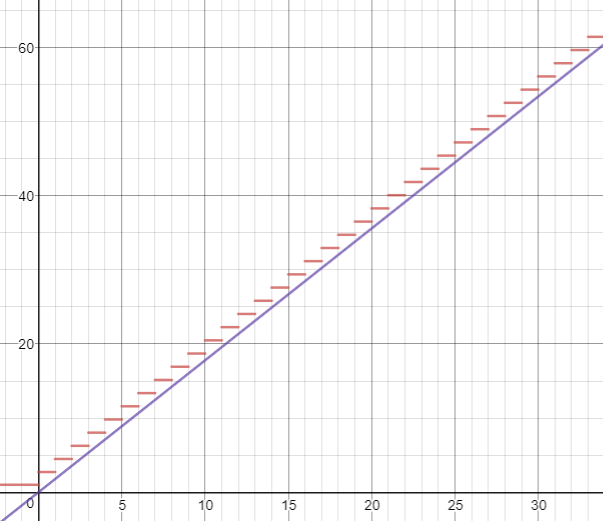# Problems of the Week

Contribute a problemGiven a cube with edge length $l$ and mass $M,$ what is the moment of inertia about the space diagonal?

$f(x) = \exp \left(\sum_{k=1}^{\lceil{x}\rceil} \frac 1k \right)$ The graph of the above is drawn in red, where $\exp(x) = e^x.$ The purple line is a linear function of the form $y=mx.$ The value of $m$ is maximized such that the purple line never intersects one of the red segments.

What is $\lfloor 1000m \rfloor ?$The Fibonacci sequence can be defined with induction as $F_n=F_{n-1}+F_{n-2}$, where $F_1=0$ and $F_2=1$. It is also well-known that the limit of the ratio between two consecutive terms $\lim \frac{F_{n+1}}{F_n}$ is the golden ratio $\phi$.

In the generalized Fibonacci sequence below, the original Fibonacci sequence is $F_n^2.$ $F_n^m=\sum_{i=1}^{m}{F_{n-i}^m}\ \text{ with }\ F_n^m=0\ \text{ if } \ n Every sequence will also have a generalized golden ratio $\phi_m=\displaystyle \lim_{n \rightarrow \infty}{ \frac{F_{n+1}^m}{F_{n}^m}}.$

What is the limit of the generalized golden ratio below? $\lim_{m \rightarrow \infty}{\phi_m} = \lim_{m \rightarrow \infty}{\lim_{n \rightarrow \infty}{\frac{F_{n+1}^m}{F_{n}^m}}}$

Three $6$-sided fair dice are rolled and their sum is recorded. If the three dice show all different values, then stop. Otherwise, roll any dice with the same value again, and add the sum of these re-rolled dice to the previous sum. Continue this process until all three dice show different values, and let $X$ be the final total of the values of all the dice rolled.

Here is an example:

• Roll 1: $(5,5,3)$ are thrown, for a running total of $13.$
• Roll 2: The two dice showing $5$ are rolled again and come up $(3,1),$ for a running total of $13+4=17.$ The three dice now show $(3,1,3).$
• Roll 3: The two dice showing $3$ are rolled again and come up $(1,1),$ for a running total of $17+2=19.$ The three dice now show $(1,1,1).$
• Roll 4: All three dice are rolled again and come up $(5,5,6),$ for a running total of $19+16=35.$
• Roll 5: The two dice showing $5$ are rolled again and come up $(1,5),$ for a running total of $35+6=41.$ The three dice now show $(1,5,6),$ so we stop with $X=41.$

The expected value of $X$ can be written as $\frac{a}{b},$ where $a$ and $b$ are coprime positive integers.

What is $a+b?$

This problem was inspired by a previous featured problem. In this version, all of the dice must show different numbers (not just the numbers that were just rolled).

A point is picked uniformly at random from the surface of an $n$-dimensional unit hypercube centered at the origin.

What is the minimum $n$ for which the expected distance from the point to the origin is greater than 1?

×